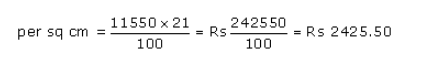# A solid is composed of a cylinder with hemispherical ends

A solid is composed of a cylinder with hemispherical ends. If the whole length of the solid is 105 cm and the diameter of the hemispherical ends is 35 cm, find the cost of polishing the surface of the solid at the rate of 21 paise per sq cm.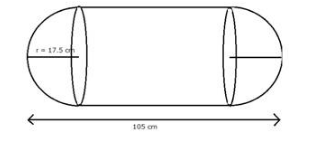Total length of the solid = 105 cm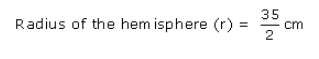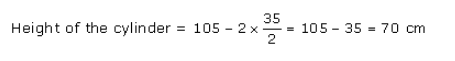Total surface area of the solid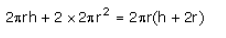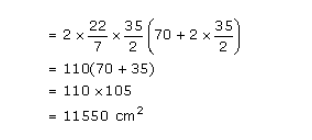Total cost of polishing the surface at the rate of 21 paise